Next: Estimation of the unmixing Up: Maximum Likelihood estimation Previous: Maximum Likelihood estimation

## Model order selection

The maximum likelihood solutions given in equations 5-7 depend on knowledge of the latent dimensionality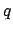. In the noise free case this quantity can easily be deduced from the rank of the covariance of the observations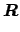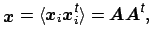which is of rank. In the presence of isotropic noise, however, the covariance matrix of the observations will be the sum of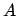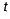and the noise covariance [Everson and Roberts, 2000]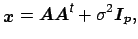(8)

i.e.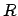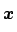will be of full rank where the additional noise has the effect of raising the eigenvalues of the covariance matrix by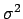. Inferring the latent dimensionality amounts to identifying the number of identical eigenvalues. In practice, however, the mismatch between the model assumption and the structure of real FMRI data renders the observed eigenspectrum to be less well behaved and we need a statistical test for the equality of eigenvalues beyond a certain threshold [Hansen et al., 1999]. Determining a cutoff value for the eigenvalues using simplistic criteria like the reconstruction error or predictive likelihood will naturally predict that the accuracy steadily increases with increased dimensionality and as such cannot be used to infer latent dimensionality. Thus, criteria like retaining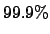of the variability result in arbitrary threshold levels that bear no relevance to the problem of estimating the latent dimensionality correctly.

Many other informal methods have been proposed, the most popular choice being the "scree plot" where one looks for a "knee" in the plot of ordered eigenvalues that signifies a split between significant and presumably unimportant directions of the data. With real FMRI data, however, the decision as to where to choose the cutoff value is not obvious and a choice based on simple visual inspection will be ambiguous (see figure 9(ii) for an example). This problem is intensified by the fact that the data set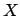is finite and thusis being estimated by the sample covariance of the set of observations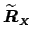. Even in the absence of any source signals, i.e. whencontains a finite number of samples from purely Gaussian isotropic noise only, the eigenspectrum of the sample covariance matrix is not identical tobut instead distributed around the true noise covariance: the eigenspectrum will depict an apparent difference in the significance of individual directions within the noise [Everson and Roberts, 2000].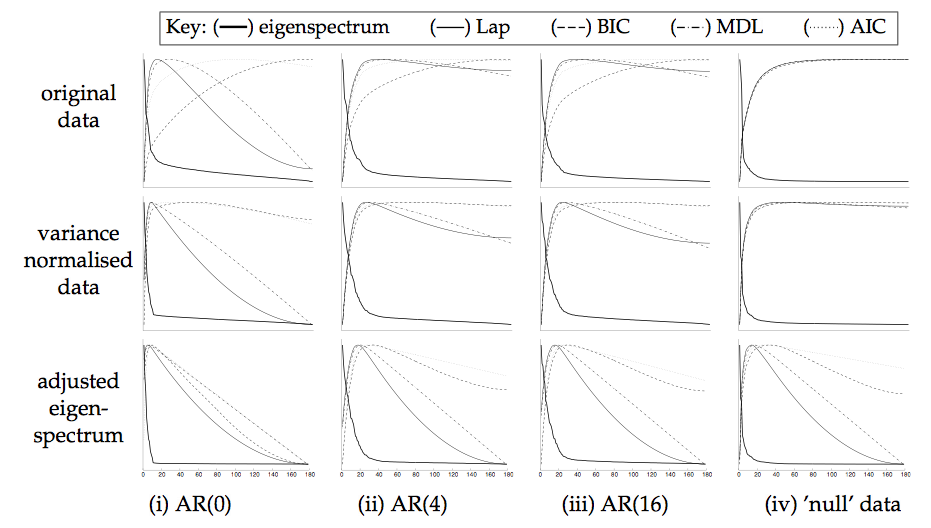In the case of purely Gaussian noise, however, the sample covariance matrixhas a Wishart distribution and we can utilise results from random matrix theory on the empirical distribution function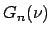for the eigenvalues of the covariance matrix of a single random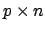-dimensional matrix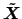[Johnstone, 2000]. Suppose that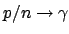as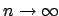and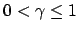, then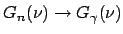almost surely, where the limiting distribution has a density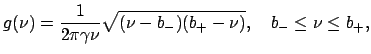(9)

and where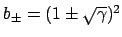. This can be used to obtain a modification to the standard scree-plot where one compares the eigenspectrum of the observations against the quantiles of the predicted cumulative distribution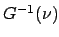, i.e. against the expected eigenspectrum of a random Gaussian matrix. The predicted eigenspectrum of the noise becomes a function of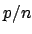: the larger, the more spread the eigenspectrum. Note that equation 9 is only satisfied for, i.e. when the number of samples is equal or larger than the dimensionality of the problem at hand. This approach is similar to [Rowe, 2001], where an inverse Wishart prior is placed on the noise covariance matrix in a fully Bayesian source separation model.

If we assume that the source distributions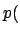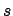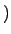are Gaussian, the probabilistic ICA model (equation 2) reduces to the probabilistic PCA model [Tipping and Bishop, 1999]. In this case, we can use more sophisticated statistical criteria for model order selection. [Minka, 2000] placed PPCA in the Bayesian framework and presented a Laplace approximation to the posterior distribution of the model evidence that can be calculated efficiently from the eigenspectrum of the covariance matrix of observations. When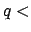min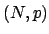, then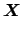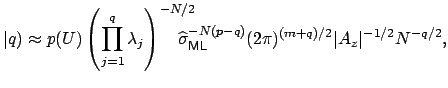(10)

where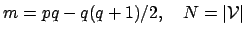and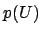denotes a uniform prior over all eigenvector matrices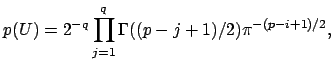and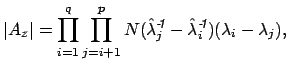where the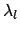denote the entries inand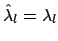forand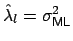otherwise. As our estimate for the latent dimensionality of the data, we choose the value ofthat maximises the approximation to the model evidence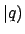.

In order to account for the limited amount of data, we combine this estimate with the predicted cumulative distribution and replaceby its adjusted eigenspectrum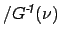prior to evaluating the model evidence. Other possible choices for model order selection for PPCA include the Bayesian Information Criterion (BIC, [Kass and Raftery, 1993]) the Akaike Information Criterion (AIC, [Akaike, 1969]) or Minimum Description Length (MDL, [Rissanen, 1978]).

Note that the estimation of the model order in the case of the probabilistic PCA model is based on the assumption of Gaussian source distribution. [Minka, 2000], however, provides some empirical evidence that the Laplace approximation works reasonably well in the case where the source distributions are non-Gaussian. As an example, figure 2 shows the eigenspectrum and different estimators of the intrinsic dimensionality for different artificial data sets, where 10 latent sources with non-Gaussian distribution were introduced into simulated AR data (i.e. auto-regressive noise where the AR parameters were estimated from real resting state FMRI data) and real FMRI resting state noise at peak levels of between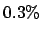and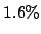of the mean signal intensity. Note how the increase in AR order will increase the estimates of the latent dimensionality, simply because there are more eigenvalues that fail the sphericity assumption. Performing variance-normalisation and adjusting the eigenspectrum usingin all cases improves the estimation. In the case of Gaussian white noise the model assumptions are correct and the adjusted eigenspectrum exactly matches equation 8. In most cases, the different estimators give similar results once the data were variance normalised and the eigenspectrum was adjusted using. Overall, the Laplace approximation and the Bayesian Information Criterion appear to give consistent estimates of the latent dimensionality even though the distribution of the embedded sources are non-Gaussian.Next: Estimation of the unmixing Up: Maximum Likelihood estimation Previous: Maximum Likelihood estimation
Christian F. Beckmann 2003-08-05